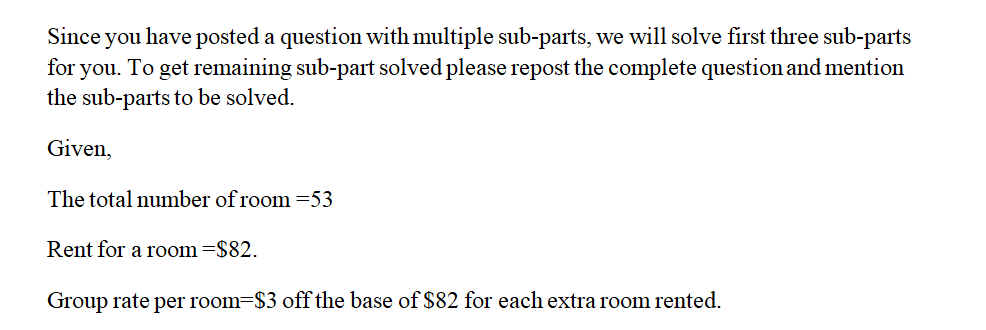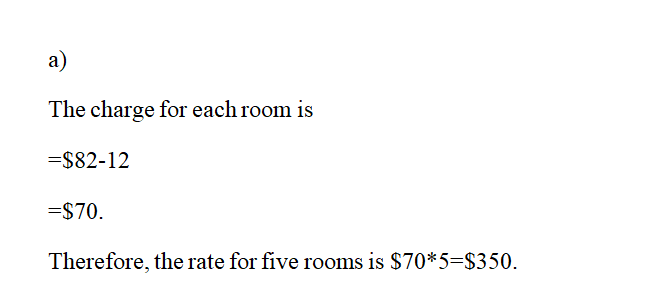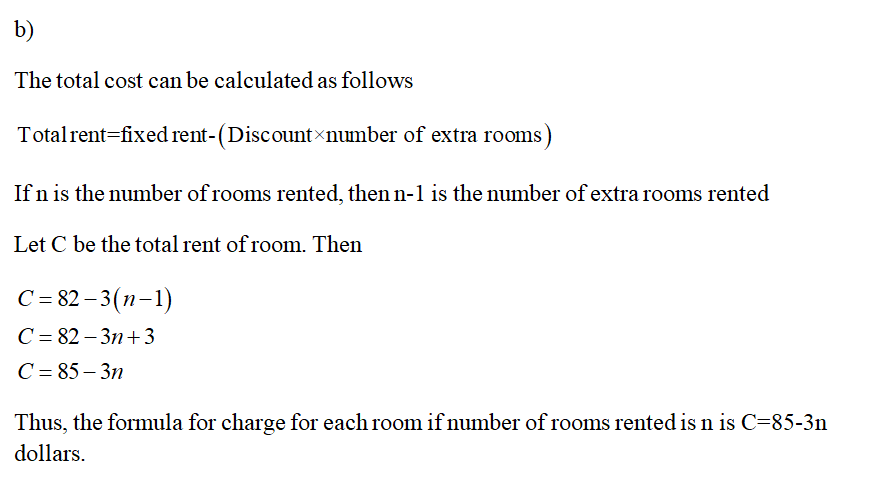# You own a motel with 53 rooms and have a pricing structure that encourages rentals of rooms in groups. One room rents for \$82, two rent for \$79 each, and in general the group rate per room is found by taking \$3 off the base of \$82 for each extra room rented.(a) How much money do you take in if a family rents five rooms? (Round your answer to the nearest dollar.)\$  (b) Use a formula to give the rate you charge for each room if you rent n rooms to an organization.    (c) Find a formula for a function R = R(n) that gives the revenue from renting n rooms to a convention host.R(n) =     (d) What is the most money you can make from rental to a single group? (Round your answer to the nearest dollar.)\$  How many rooms do you rent?

Question
1 views

You own a motel with 53 rooms and have a pricing structure that encourages rentals of rooms in groups. One room rents for \$82, two rent for \$79 each, and in general the group rate per room is found by taking \$3 off the base of \$82 for each extra room rented.

(a) How much money do you take in if a family rents five rooms? (Round your answer to the nearest dollar.)
\$

(b) Use a formula to give the rate you charge for each room if you rent n rooms to an organization.

(c) Find a formula for a function R = R(n) that gives the revenue from renting n rooms to a convention host.
R(n) =

(d) What is the most money you can make from rental to a single group? (Round your answer to the nearest dollar.)
\$

How many rooms do you rent?

check_circle

Step 1Step 2Step 3...

### Want to see the full answer?

See Solution

#### Want to see this answer and more?

Solutions are written by subject experts who are available 24/7. Questions are typically answered within 1 hour.*

See Solution
*Response times may vary by subject and question.
Tagged in

### Algebra